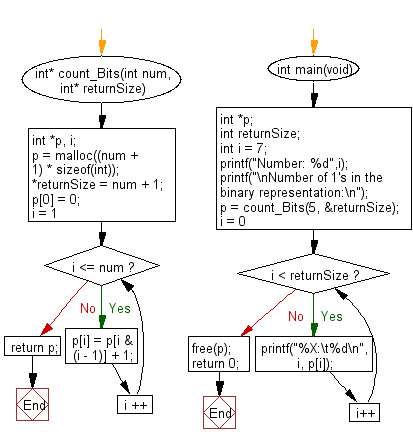﻿ C Program: 1 in their binary representation as an array

# C Exercises: Calculate the number of 1's in their binary representation and return them as an array

## C Programming Mathematics: Exercise-14 with Solution

For a non negative integer in the range 0 ≤ i ≤ n write a C program to calculate the number of 1's in their binary representation and return them as an array.

Example:
Input:
Number: 7
Number of 1's in the binary representation:
0: 0
1: 1
2: 1
3: 2
4: 1
5: 2

Sample Solution:

C Code:

``````#include <stdio.h>
#include <stdlib.h>

int* count_Bits(int num, int* returnSize) {
int *p, i;

p = malloc((num + 1) * sizeof(int));
*returnSize = num + 1;

p = 0;
for (i = 1; i <= num; i ++) {
p[i] = p[i & (i - 1)] + 1;
}

return p;
}

int main(void)
{
int *p;
int returnSize;
int i = 7;
printf("Number: %d",i);
printf("\nNumber of 1's in the binary representation:\n");
p = count_Bits(5, &returnSize);
for (i = 0; i < returnSize; i++) {
printf("%X:\t%d\n", i,  p[i]);
}
free(p);
return 0;
}
```
```

Sample Output:

```Number: 7
Number of 1's in the binary representation:
0:	0
1:	1
2:	1
3:	2
4:	1
5:	2
```

Flowchart:C Programming Code Editor:

Improve this sample solution and post your code through Disqus.

What is the difficulty level of this exercise?

Test your Programming skills with w3resource's quiz.

﻿